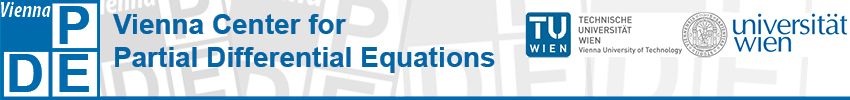## Vienna PDE Day

The aim of the event is to intensify the cooperation of the PDE groups in Vienna. The scientific talks shall help to identify possible links between different research fields on PDEs and related topics. Emphasis will be given on nontechnical presentations which point out possible connections and open questions. There will be plenty of time for discussions during the breaks (lunch buffet will be provided).

Time: June 16, 2015, 9:00h - 17:30h

Location: TUtheSky, 11th floor, Getreidemarkt 9, 1060 Wien

Speakers:

• Mathias Beiglböck (Uni Wien)
A systematic approach to Skorokhod embedding
The Skorokhod embedding problem is to represent a given probability as the distribution of Brownian motion at a chosen stopping time. Over the last 50 years this has become one of the important classical problems in probability theory and a number of authors have constructed solutions with particular optimality properties, based on different tools from stochastic analysis. Very recently a new systematic method to construct optimal embeddings has been established: as a first step a geometric description is obtained through mass transport techniques. A complete characterization can then be obtained using PDEs. This allows us, for the first time, to derive all known optimal embeddings as special cases of one unified construction and leads to a variety of new embeddings.
• Francesca Bonizzoni (Uni Wien)
PDEs with stochastic data
Mathematical models are helpful instruments for qualitative and quantitative investigation. In many applications the parameters of the model are not precisely known, either because they involve quantities which can be measured only point-wise or because they are intrinsically variable. Recently, many efforts have been done in trying to treat and include this uncertainty in the model. A very convenient framework is offered by the probability theory. Starting from a suitable PDE model, the uncertain input data are described as random variables or random fields with known probability laws. This kind of mathematical models is called stochastic PDE (SPDE). The solution of an SPDE is itself stochastic. The goal of the Uncertainty Quantification is to infer on the solution, i.e. to understand how the uncertainty in the input data of the model reflects onto the solution. The quantities of interest may be the statistics of the solution itself, or statistics of functionals of the solution. The aim of the talk is to infer on the statistical moments of the solution.
• Eric Carlen (Rutgers University, USA)
Non-equilibrium steady state, and approach to them, for the thermostatted kinetic Fokker-Planck equation
We consider a class of non-spatially homogeneous kinetic equations describing a gas interacting with heat reservoirs at different temperatures, including a kinetic Fokker-Plank equation. We prove existence and uniqueness of steady states in a variety of such models, and exponential relaxation to the steady state for all reasonable data, not only data close to equilibrium. This is joint work with Joel Lebowitz and Clement Mouhot.
• Markus Melenk (TU Wien)
High order methods for high-frequency wave problems
The simulation of acustic or electromagnetic wave problems is often based on the Helmholtz equation. We discuss two approaches to this problem class. First, we analyze high order FEM discretizations and show that quasi-optimality can be achieved if the approximation order p is chosen as p = O(log k). In the context of a 2D boundary element method (BEM), we show that specialized methods that build the known asymptotics of the solution into the ansatz space can achieve a fixed accuracy with work almost independent of k.
• Laurent Pfeiffer (Uni Graz)
Optimal control of the Fokker-Planck equation
This talk is devoted to optimal control problems of stochastic differential equations, where the cost function depends on the probability distribution of the final state. This distribution is the solution to the Fokker-Planck equation, a parabolic partial differential equation. We derive optimality conditions and suggest an iterative numerical method which is based on a linearization of the problem, for which the usual semi-Lagrangian schemes can be used.
• Joachim Schöberl (TU Wien)
Computational Fluid Dynamics - From Boltzmann to Euler and Navier Stokes
In this talk we discuss several modern discretization techniques in fluid dynamics: Hybrid Discontinuous Galerkin methods for incompressible Navier Stokes Equations and space-time methods for compressible Euler Equations. Traditionally, the macroscopic equations are obtained by a hierarchical expansion of the Boltzmann equation in momentum space. We present our recent research, where this expansion is performed on the numerical level. This allows to combine very naturally different models in different spatial regions. Furthermore, the interpretation as a kinetic equation gives also a deeper understanding of methods for the macroscopic equations. We present several numerical examples simulated with our parallel finite element software NGSolve.
• Ulisse Stefanelli (Uni Wien)
Existence results in finite plasticity
A classical approach to finite plastic deformations prescribes the deformation gradient grad(φ) of the medium to be multiplicatively decomposed as ∇φ = Fe Fp, where Fe stands for the elastic and Fp for the plastic strain, respectively. This decomposition is a fundamental source of nonlinearities as thee elastic energy is given in terms of Fe = ∇φFp-1 only. In addition, dissipation dynamics are also nonlinear, as the plastic strain is usually restricted to have determinant 1. Correspondingly, existence results for quasistatic evolution in finite plasticity in this context are just a few. I shall present the basics of the available theories and comment on some recent development. In particular, I will mention the possibility of rigorously ascertain the small-deformation, linear limit in terms of evolutive Γ-convergence techniques.

Schedule:

 9:00 - 9:50 Eric Carlen 9:55 - 10:45 Ulisse Stefanelli 10:50 - 11:20 Coffee break 11:20 - 12:10 Laurent Pfeiffer 12:15 - 13:30 Lunch buffet 13:30 - 14:20 Francesca Bonizzoni 14:25 - 15:15 Mathias Beiglböck 15:20 - 15:50 Coffee break 15:50 - 16:40 Markus Melenk 16:45 - 17:35 Joachim Schöberl